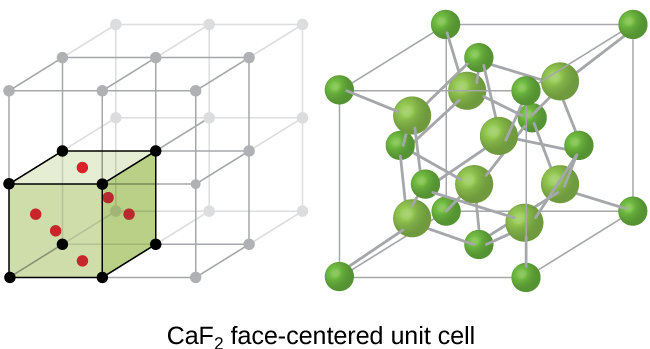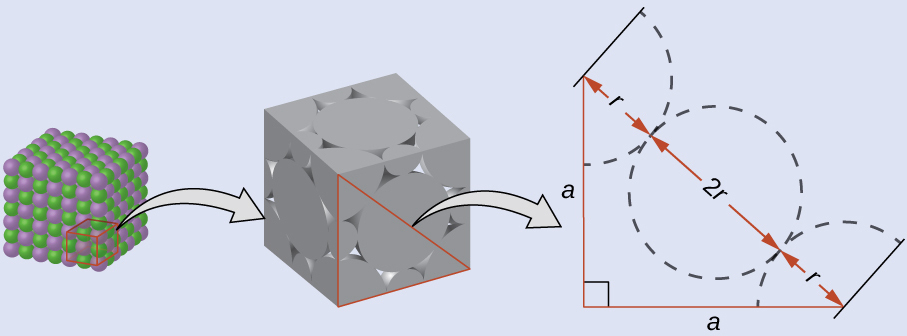# 10.6 Lattice structures in crystalline solids  (Page 8/29)

 Page 8 / 29Calcium fluoride, CaF 2 , forms an FCC unit cell with calcium ions (green) at the lattice points and fluoride ions (red) occupying all of the tetrahedral sites between them.

If we know the edge length of a unit cell of an ionic compound and the position of the ions in the cell, we can calculate ionic radii for the ions in the compound if we make assumptions about individual ionic shapes and contacts.

The edge length of the unit cell of LiCl (NaCl-like structure, FCC) is 0.514 nm or 5.14 Å. Assuming that the lithium ion is small enough so that the chloride ions are in contact, as in [link] , calculate the ionic radius for the chloride ion.

Note: The length unit angstrom, Å, is often used to represent atomic-scale dimensions and is equivalent to 10 −10 m.

## Solution

On the face of a LiCl unit cell, chloride ions contact each other across the diagonal of the face:Drawing a right triangle on the face of the unit cell, we see that the length of the diagonal is equal to four chloride radii (one radius from each corner chloride and one diameter—which equals two radii—from the chloride ion in the center of the face), so d = 4 r . From the Pythagorean theorem, we have:

${a}^{2}+{a}^{2}={d}^{2}$

which yields:

${\left(0.514\phantom{\rule{0.2em}{0ex}}\text{nm}\right)}^{2}+{\left(0.514\phantom{\rule{0.2em}{0ex}}\text{nm}\right)}^{2}={\left(4r\right)}^{2}=16{r}^{2}$

Solving this gives:

$r=\phantom{\rule{0.2em}{0ex}}\sqrt{\frac{{\left(0.514\phantom{\rule{0.2em}{0ex}}\text{nm}\right)}^{2}+{\left(0.514\phantom{\rule{0.2em}{0ex}}\text{nm}\right)}^{2}}{16}}\phantom{\rule{0.2em}{0ex}}=\text{0.182 nm}\phantom{\rule{0.2em}{0ex}}\left(\text{1.82 Å}\right)\phantom{\rule{0.2em}{0ex}}{\text{for a Cl}}^{\text{−}}\phantom{\rule{0.2em}{0ex}}\text{radius}.$

The edge length of the unit cell of KCl (NaCl-like structure, FCC) is 6.28 Å. Assuming anion-cation contact along the cell edge, calculate the radius of the potassium ion. The radius of the chloride ion is 1.82 Å.

The radius of the potassium ion is 1.33 Å.

It is important to realize that values for ionic radii calculated from the edge lengths of unit cells depend on numerous assumptions, such as a perfect spherical shape for ions, which are approximations at best. Hence, such calculated values are themselves approximate and comparisons cannot be pushed too far. Nevertheless, this method has proved useful for calculating ionic radii from experimental measurements such as X-ray crystallographic determinations.

## X-ray crystallography

The size of the unit cell and the arrangement of atoms in a crystal may be determined from measurements of the diffraction of X-rays by the crystal, termed X-ray crystallography    . Diffraction is the change in the direction of travel experienced by an electromagnetic wave when it encounters a physical barrier whose dimensions are comparable to those of the wavelength of the light. X-rays are electromagnetic radiation with wavelengths about as long as the distance between neighboring atoms in crystals (on the order of a few Å).

When a beam of monochromatic X-rays strikes a crystal, its rays are scattered in all directions by the atoms within the crystal. When scattered waves traveling in the same direction encounter one another, they undergo interference , a process by which the waves combine to yield either an increase or a decrease in amplitude (intensity) depending upon the extent to which the combining waves’ maxima are separated (see [link] ).

what is the meaninh of link
Yes wish to join am a science ⚗ student
What is acid
Emmanuel
is agroup of substance that relaise hydrogen ion in the water solution.
mengistu
thanks
Aisha
how can someone understand chemistry vividly
Maybe by reading proofs or practical work and application in modern world.
Abdelkarim
what is isotopes
whats de shape of water
water has no shape because it's liquid
Wil
water is a shapeless, odourless, colourless and tasteless substance that only takes the shape of its container.
mikefred
i think they're referring to the molecular shape?
It has no shape but takes the shape of the container
what isthe maening pkw
what is the meaning of STP
Laura
introduction to chemistry for beginner
hi
noble
through out human history
Yes?
Esther
hello
noble
yes
Bol
what
noble
is euglena a unicellular organ
is euglena a unicellular organism
Agio
how is hydrogen can be heated
what is difference between atom and molecule
Atom is the smallest part of matter; it consists of equal number of protons and electrons. It may have neutrons. A molecule is a compound made of atoms covalently bonded.
Abdelkarim
does amoeba has structure
what is the effect of green house on the earth 🌎
what is the effect of green house on the earth 🌎
kolawole
what's an atom?
it's the smallest unit of Matter
Orsine
atom
Eden
smallest part of an element
lydia
also, depending on its (atom's) structure, that is the amount of protons and neutrons and electrons, is the determining factors of what element it is.
Richard
is a smallast particals of an element
Buwembo
it is the smallest part of an element that can take part in a chemical reaction
Ayub
is the smallest part of an element
Jonathan
An atom is the smallest indivisible part of a matter
Oy kl konsa test hay or kitna hay?

#### Get Jobilize Job Search Mobile App in your pocket Now!ByByBy Yasser IbrahimBy Robert MurphyBy Rohini AjayBy OpenStaxBy OpenStaxBy Nicole DuquetteBy OpenStaxBy Madison ChristianBy Janet ForresterBy Danielrosenberger